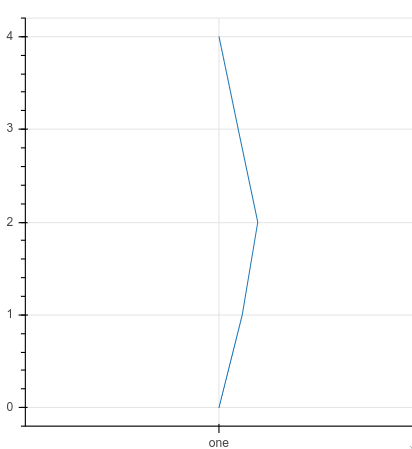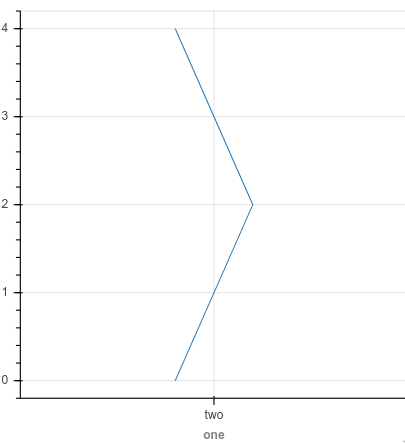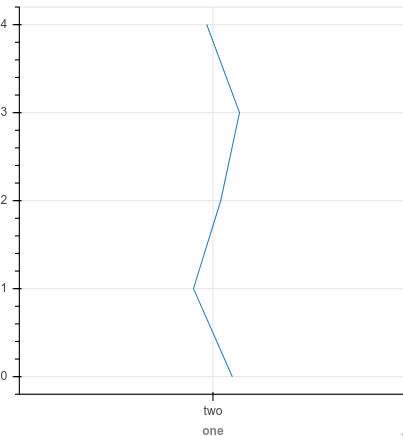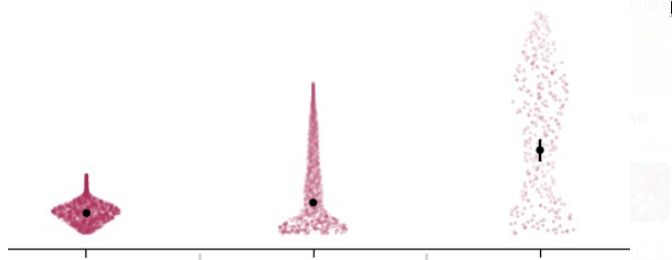# How can I plot a line with an offset along a nested categorical axes?

Plotting a line with an x offset works with categorical axes:

``````from bokeh.plotting import figure, show
from bokeh.io import output_notebook

output_notebook()

ys = list(range(5))
line_xs = [0, 0.06, 0.1, 0.05, 0]
cat_xs = ['one']

p = figure(width=400, height=400, x_range=cat_xs)
# p.circle([cat_xs], 1)
p.line([(x, l) for x, l in zip(cat_xs * len(ys), line_xs)], ys)

show(p)
``````However, when trying it with a nested categorical, there is no output in the notebook, and the js console shows an error:

``````from bokeh.models import FactorRange

ys = list(range(5))
line_xs = [0, 0.06, 0.1, 0.05, 0]
cat_xs = [('one', 'two')]

p = figure(width=400, height=400, x_range=FactorRange(*cat_xs))
# p.circle([cat_xs], 1)
p.line([(x, l) for x, l in zip(cat_xs * len(ys), line_xs)], ys)

show(p)
``````
``````VM2620:382 Uncaught TypeError: (intermediate value)(intermediate value)(intermediate value) is not iterable
at _ (<anonymous>:382:2281)
at _ (<anonymous>:382:2294)
at Object.t.decode_column_data (<anonymous>:382:3285)
at p.initialize (<anonymous>:355:1394)
at p.finalize (<anonymous>:289:2698)
at <anonymous>:276:6267
at o (<anonymous>:276:6045)
at o (<anonymous>:276:6037)
at o (<anonymous>:276:6093)
at o (<anonymous>:276:6037)
``````

One way I found that works is to assume that the first category is at 0.5, and then give points around this, e.g. `p.line([0.4, 0.5, 0.6, 0.5, 0.4], ys)`. Is this a safe assumption? Are bokeh categories always at 0.5, 1.5 etc? Or is there a better way to do this (probably)?``````# Versions
-----
bokeh       2.0.2
sinfo       0.3.1
-----
IPython             7.13.0
jupyter_client      6.1.3
jupyter_core        4.6.3
jupyterlab          1.2.6
notebook            6.0.3
-----
Python 3.8.3 (default, May 19 2020, 18:47:26) [GCC 7.3.0]
Linux-4.15.0-101-generic-x86_64-with-glibc2.10
``````

I tried some more and realized that using a CDS might work together with a transform, but I can’t find a way to control to offset per point, either I can use `jitter` to have a different but random offset per point (as shown below), or I can use `dodge` to have a uniform offset per point. I want to specify an array of points indicating the offset per node of the line, is this possible

``````from bokeh.models import ColumnDataSource
from bokeh.transform import jitter

ys = list(range(5))
line_xs = [0, 0.06, 0.1, 0.05, 0]
cat_xs = [('one', 'two')]
cds = ColumnDataSource(data={'ys': ys, 'line_xs': line_xs, 'dummy': [0.5]*5})

p = figure(width=400, height=400, x_range=FactorRange(*cat_xs))
x_jitter = jitter('dummy', 0.2, range=p.x_range)
p.line(x_jitter, 'ys', source=cds)

show(p)
``````I noticed that there is a custom JS transform, which I am guessing could do what I wanted if I was proficient in JS. My use case is to have the line approximate the density of the data (to create a violin or ridge plot), and I don’t know how I would implement this with a custom JS transform (maybe something like this). Ideally, if there was a way to pass the array of offset point (computed via scipy/statsmodel KDE) and then just write the custom JS transform to apply each value that would be great, but from the example in the docs I don’t understand how I can pass a precomputed array to the js transform, it looks like it doesn’t take any arguments (but I might just be misunderstanding).

I tried patch also as in the ridgeplot example in the gallery, but had the same issue, I don’t think a band will work since it is an annotation and I want the line to show up above my data (a violinplot outline on top of plotted circles).

This is true unless you start configuring the various padding properties manually.

I don’t have time just now to try and update your code but a quick look at the data you are passing:

``````[(('one', 'two'), 0),
(('one', 'two'), 0.06),
(('one', 'two'), 0.1),
(('one', 'two'), 0.05),
(('one', 'two'), 0)]
``````

Looks wrong to me. IIRC it should just be `[('one', two', 0), ('one', 'two', 0.06), .. ]` i.e the offset and the factors should all be at the same level.

Ah well spotted! Thanks for solving yet another of my issues @Bryan ! I really appreciate the responsiveness from the Bokeh team at these forums.

Finding out about the nested category offset syntax allowed me to lay out points according to density estimates which is working really well for my application! (Also the webgl bokeh integration is fantastic, so smoooooth!)1 Like

This would be a great example for the gallery if you are ever interested to make a new contribution!

Thanks, I would love to contribute back! I will ping you when I make a PR (might be a little while). For the example I’m imagining that sampling from a few numpy distributions would be a good way to illustrate the behavior of this plot (maybe bimodal, random, normal with a couple of SD s, and skewed/long tailed/power law). Let me know if you have another preference.